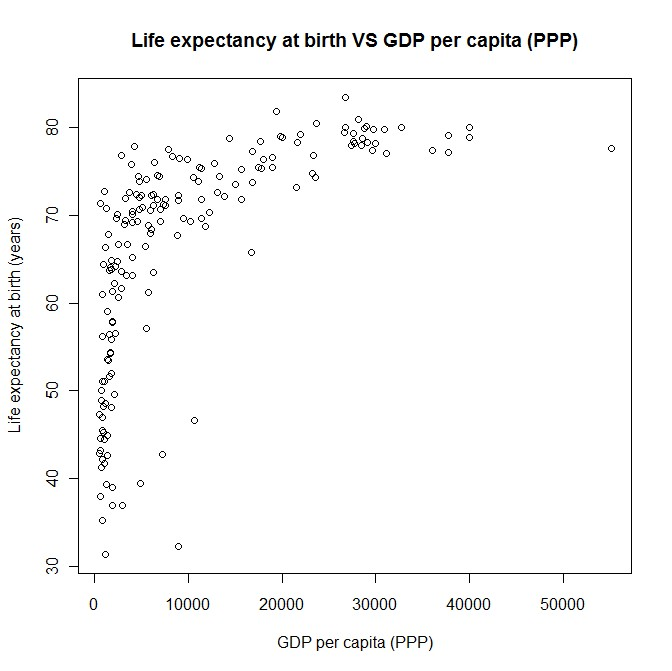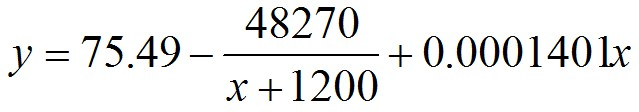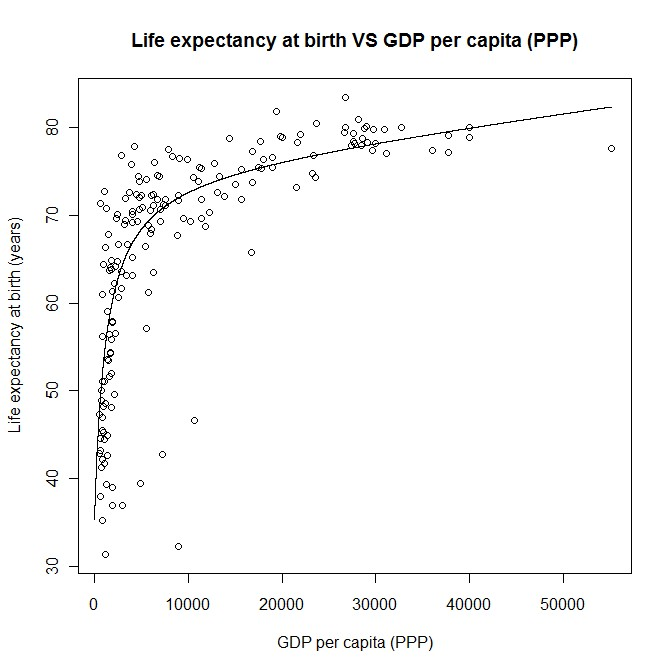# Life expectancy at birth versus GDP per capita (PPP)

Graphs / Images, Econometrics / EconomicsThe data for this graph is available from the Index Mundi website.  The data is from 2003.

The graph shows that life expectancy at birth, increases at a decreasing rate with respect to GDP per capita (PPP).

The main reason for this non-linear relationship is because people consume both needs and wants.  People consume needs in order to survive.  Once a person’s needs are satisfied, they could then spend the rest of their money on non-necessities.  If everyone’s needs are satisfied, then any increase in GDP per capita would barely affect life expectancy.

GDP per capita isn't the only thing that affects life expectancy.  Government intervention can also affect it.  A nation could be rich, but if its government ignores the plight of the poor, it could lower the life expectancy.

Another reason for the wide variation in the life expectancies for countries with low GDP per capita would be due to the level of non-market economic activity.  For example, if there is a lot of subsistence farming, people could be working and have enough food to eat, but wouldn’t be contributing much to the nation’s GDP because they wouldn’t be buying the food they eat, or selling the food they grow i.e. no exchange of money.

The relationship between life expectancy and GDP per capita is strong enough to be the basis of a regression model.  Simple functions that increase at a decreasing rate include multiplicative (hyperbolas) and logarithmic functions.

The following is R output for a regression model that was fitted to the data:

> fit=lm(LifeExpectancyAtBirth~I(1/(GDPpcPPP+1200))+GDPpcPPP+LElt40)
> summary(fit)

Call:
lm(formula = LifeExpectancyAtBirth ~ I(1/(GDPpcPPP + 1200)) +
GDPpcPPP + LElt40)

Residuals:
Min       1Q   Median       3Q      Max
-27.9828  -2.5941   0.3209   3.0735  22.6424

Coefficients:
Estimate Std. Error t value Pr(>|t|)
(Intercept)             7.549e+01  1.513e+00  49.884   <2e-16 ***
I(1/(GDPpcPPP + 1200)) -4.827e+04  4.376e+03 -11.032   <2e-16 ***
GDPpcPPP                1.401e-04  6.537e-05   2.143   0.0335 *
LElt40                 -2.319e+01  2.200e+00 -10.540   <2e-16 ***
---
Signif. codes:  0 ‘***’ 0.001 ‘**’ 0.01 ‘*’ 0.05 ‘.’ 0.1 ‘ ’ 1

Residual standard error: 6.316 on 176 degrees of freedom
Multiple R-squared: 0.7591,     Adjusted R-squared: 0.7549
F-statistic: 184.8 on 3 and 176 DF,  p-value: < 2.2e-16

The above output won’t make much sense to a layperson.  The regression output shows that the following equation was estimated (for countries with life expectancy of at least 40):where   y is the life expectancy at birth
x is GDP per capita (PPP)

The regression model includes:
• An intercept
• A hyperbolic term
• A linear term
• A dummy variable with a 1 for countries with a life expectancy less than 40; 0 otherwise.  There were only nine countries with life expectancy less than 40.
The model fits quite well to the data (R-squared statistic of 75.9%).  It isn’t necessarily the best model, but it appears to be a fairly good one.

The estimated equation can be added to the graph: